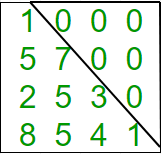Related Articles

# Program to check if matrix is lower triangular

• Difficulty Level : Basic
• Last Updated : 26 Apr, 2021

Given a square matrix and the task is to check the matrix is in lower triangular form or not. A square matrix is called lower triangular if all the entries above the main diagonal are zero.Examples:

```Input : mat = {{1, 0, 0, 0},
{1, 4, 0, 0},
{4, 6, 2, 0},
{0, 4, 7, 6}};
Output : Matrix is in lower triangular form.

Input : mat = {{1, 0, 0, 0},
{4, 3, 0, 1},
{7, 9, 2, 0},
{8, 5, 3, 6}};
Output : Matrix is not in lower triangular form.```

## C++

 `// Program to check lower``// triangular matrix.``#include ``#define N 4``using` `namespace` `std;` `// Function to check matrix is in``// lower triangular form or not.``bool` `isLowerTriangularMatrix(``int` `mat[N][N])``{``    ``for` `(``int` `i = 0; i < N; i++)``        ``for` `(``int` `j = i + 1; j < N; j++)``            ``if` `(mat[i][j] != 0)``                ``return` `false``;``    ``return` `true``;``}` `// Driver function.``int` `main()``{``    ``int` `mat[N][N] = { { 1, 0, 0, 0 },``                      ``{ 1, 4, 0, 0 },``                      ``{ 4, 6, 2, 0 },``                      ``{ 0, 4, 7, 6 } };` `    ``// Function call``    ``if` `(isLowerTriangularMatrix(mat))``        ``cout << ``"Yes"``;``    ``else``        ``cout << ``"No"``;``    ``return` `0;``}`

## Java

 `// Java Program to check for``// a lower triangular matrix.``import` `java.io.*;` `class` `Lower_triangular``{``    ``int` `N = ``4``;` `    ``// Function to check matrix is``    ``// in lower triangular form or not.``    ``boolean` `isLowerTriangularMatrix(``int` `mat[][])``    ``{``        ``for` `(``int` `i = ``0``; i < N; i++)``            ``for` `(``int` `j = i + ``1``; j < N; j++)``                ``if` `(mat[i][j] != ``0``)``                    ``return` `false``;` `        ``return` `true``;``    ``}` `    ``// Driver function.``    ``public` `static` `void` `main(String args[])``    ``{``        ``Lower_triangular ob = ``new` `Lower_triangular();``        ``int` `mat[][] = { { ``1``, ``0``, ``0``, ``0` `},``                        ``{ ``1``, ``4``, ``0``, ``0` `},``                        ``{ ``4``, ``6``, ``2``, ``0` `},``                        ``{ ``0``, ``4``, ``7``, ``6` `} };``                        ` `        ``// Function call``        ``if` `(ob.isLowerTriangularMatrix(mat))``            ``System.out.println(``"Yes"``);``        ``else``            ``System.out.println(``"No"``);``    ``}``}` `// This code is contributed by Anshika Goyal.`

## Python3

 `# Python3 Program to check``# lower triangular matrix.` `# Function to check matrix``# is in lower triangular``def` `islowertriangular(M):``    ``for` `i ``in` `range``(``0``, ``len``(M)):``        ``for` `j ``in` `range``(i ``+` `1``, ``len``(M)):``            ``if``(M[i][j] !``=` `0``):``                    ``return` `False``    ``return` `True``    ` `# Driver function.``M ``=` `[[``1``,``0``,``0``,``0``],``     ``[``1``,``4``,``0``,``0``],``     ``[``4``,``6``,``2``,``0``],``     ``[``0``,``4``,``7``,``6``]]` `if` `islowertriangular(M):``    ``print` `(``"Yes"``)``else``:``    ``print` `(``"No"``)``    ` `# This code is contributed by Anurag Rawat`

## C#

 `// C# program to check for``// a lower triangular matrix.``using` `System;` `class` `Lower_triangular``{``    ``int` `N = 4;` `    ``// Function to check matrix is``    ``// in lower triangular form or not.``    ``bool` `isLowerTriangularMatrix(``int``[, ] mat)``    ``{``        ``for` `(``int` `i = 0; i < N; i++)``            ``for` `(``int` `j = i + 1; j < N; j++)``                ``if` `(mat[i, j] != 0)``                    ``return` `false``;` `        ``return` `true``;``    ``}` `    ``// Driver function.``    ``public` `static` `void` `Main()``    ``{``        ``Lower_triangular ob = ``new` `Lower_triangular();``        ``int``[, ] mat = { { 1, 0, 0, 0 },``                        ``{ 1, 4, 0, 0 },``                        ``{ 4, 6, 2, 0 },``                        ``{ 0, 4, 7, 6 } };` `        ``// Function call``        ``if` `(ob.isLowerTriangularMatrix(mat))``            ``Console.WriteLine(``"Yes"``);``        ``else``            ``Console.WriteLine(``"No"``);``    ``}``}` `// This code is contributed by vt_m.`

## PHP

 ``

## Javascript

 ``

Output:

`Yes`

Attention reader! All those who say programming isn’t for kids, just haven’t met the right mentors yet. Join the  Demo Class for First Step to Coding Coursespecifically designed for students of class 8 to 12.

The students will get to learn more about the world of programming in these free classes which will definitely help them in making a wise career choice in the future.

My Personal Notes arrow_drop_up